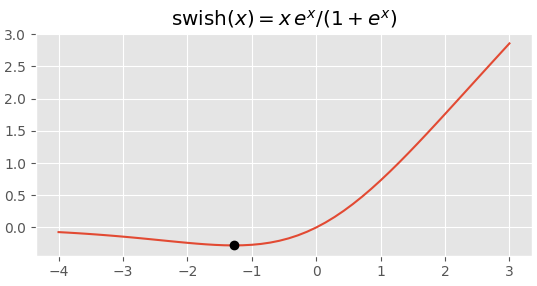# Swish function and a Swiss mathematician

The previous post looked at the swish function and related activation functions for deep neural networks designed to address the “dying ReLU problem.”Unlike many activation functions, the function f(x) is not monotone but has a minimum near x0 = -1.2784. The exact location of the minimum iswhere W is the Lambert W function, named after the Swiss mathematician Johann Heinrich Lambert .

The minimum value of f is -0.2784. I thought maybe I made a mistake, confusing x0 and f(x0). If you look at more decimal place, the minimum value of f is

-0.278464542761074

and occurs at

-1.278464542761074.

That can’t be a coincidence.

It turns out you can prove that f(x0) − x0 = 1 without explicitly finding x0. Take the derivative of f using the quotient rule and set the numerator equal to zero. This shows that at the minimum,ThenThe fourth equation is where we use the equation satisfied at the minimum.

 Lambert is sometimes considered Swiss and sometimes French. The plot of land he lived on belonged to Switzerland at the time, but now belongs to France. I wanted him to be Swiss so could use “swish” and “Swiss” together in the title.

## One thought on “Swish function and a Swiss mathematician”

1. Paul Clapham

I see that the Republic of Mulhouse didn’t become part of France until well after Lambert died, so I’d say you would be justified as describing him as “Swiss”.